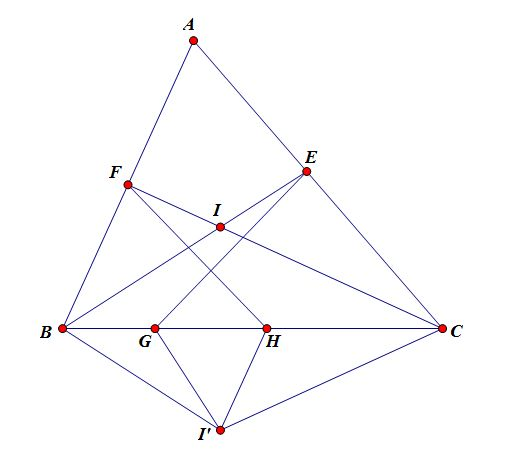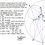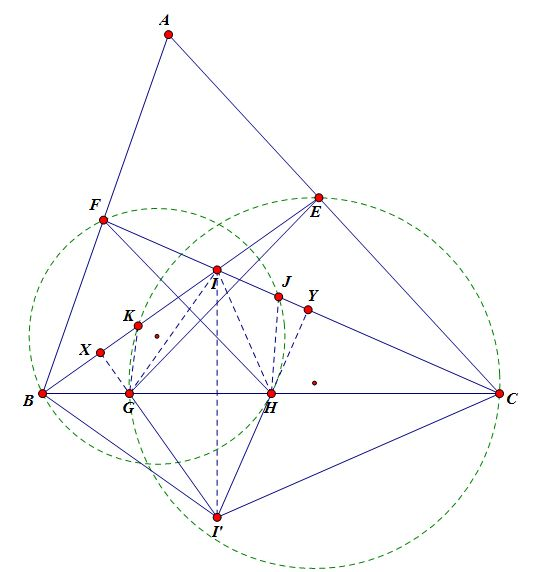# [Solutions Posted]Geometry Proof Problem of the day #4

I used to think algebraic geometry and analytic geometry are the same thing. How naive was I?Given $$\triangle ABC$$, let $$BE,CF$$ be angle bisectors such that $$E\in AC, F\in AB$$. Reflect the incenter $$I$$of $$ABC$$ over $$BC$$ to obtain $$I'$$. $$G,H\in BC$$ such that $$I'G\perp BE, I'H\perp CF$$. Prove that $$\angle FHB=\angle EGC$$Note by Xuming Liang
5 years, 10 months ago

This discussion board is a place to discuss our Daily Challenges and the math and science related to those challenges. Explanations are more than just a solution — they should explain the steps and thinking strategies that you used to obtain the solution. Comments should further the discussion of math and science.

When posting on Brilliant:

• Use the emojis to react to an explanation, whether you're congratulating a job well done , or just really confused .
• Ask specific questions about the challenge or the steps in somebody's explanation. Well-posed questions can add a lot to the discussion, but posting "I don't understand!" doesn't help anyone.
• Try to contribute something new to the discussion, whether it is an extension, generalization or other idea related to the challenge.

MarkdownAppears as
*italics* or _italics_ italics
**bold** or __bold__ bold
- bulleted- list
• bulleted
• list
1. numbered2. list
1. numbered
2. list
Note: you must add a full line of space before and after lists for them to show up correctly
paragraph 1paragraph 2

paragraph 1

paragraph 2

[example link](https://brilliant.org)example link
> This is a quote
This is a quote
    # I indented these lines
# 4 spaces, and now they show
# up as a code block.

print "hello world"
# I indented these lines
# 4 spaces, and now they show
# up as a code block.

print "hello world"
MathAppears as
Remember to wrap math in $$ ... $$ or $ ... $ to ensure proper formatting.
2 \times 3 $2 \times 3$
2^{34} $2^{34}$
a_{i-1} $a_{i-1}$
\frac{2}{3} $\frac{2}{3}$
\sqrt{2} $\sqrt{2}$
\sum_{i=1}^3 $\sum_{i=1}^3$
\sin \theta $\sin \theta$
\boxed{123} $\boxed{123}$

Sort by:

I came up with this solution with the intention to use cyclic, therefore it is different than my original solution(3 years old). Instead of posting that as solution 2, I will give out hints to allow someone else to devise the proof: Extend $I'G,I'H$ more so that they intersect $AB,AC$, how can symmetry suggest what to do next?Solution 1: Construct $(FBH)\cap CF=J\ne F, (ECG)\cap BE=K\ne E$. Then $\angle FHG=\angle EGC\iff \angle FJB=\angle EKC\iff B,C,K,J$ are concyclic.

Extend $I'G,I'H$ to meet $BI,CI$ at $X,Y$. Since $B,C,Y,X$ can be proven to be concyclic, it suffices to show that $XY||KJ$. Note that $\angle IGX=\angle IBI'=\angle B=\angle CJH$, thus $IGX\sim HJY$. Likewise we can show $IHY\sim GKX$. Hence by ratios, $JY\cdot IX=XG\cdot HY=XK\cdot IY\implies \frac {JK}{IY}=\frac {XK}{IX}\implies XY||KJ$. We are done.

- 5 years, 10 months ago

@Xuming Liang Next in the series pls? :D

- 2 years, 12 months ago

nice and easy one.

- 5 years, 10 months ago

Denote by $I'G \cap AB= K, I'H \cap AC =L.$ Then, $\angle AIF = \angle AKI = 90^{\circ} - \angle \frac{B}{2}$ $\therefore$ By PoP, $AF \times AK = AI^{2}$ Similarly, $AE \times AL = AI^{2} \Rightarrow EFKL$ is cyclic $\Rightarrow \angle EKF = \angle FLE \Longrightarrow \angle FHB =\angle EGC.$ $\blacksquare$

- 2 years, 12 months ago